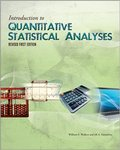## Faculty-Authored Books#### Title

Introduction to Quantitative Statistical Analyses

#### Description

Introduction to Quantitative Statistical Analyses is designed for beginning statistics courses that focus on the statistical procedures commonly used in experimental and correlational research. Following an approach taken by Keppel and his associates, the text seeks to familiarize students with the statistical tests most often encountered in the literature. Doing calculations by hand is important for understanding statistical operations, while using computer software (e.g., SPSS) is important for future statistical work. This text emphasizes both approaches, helping students develop a deeper understanding of the application of statistical analyses.

The book also explores some limitations of the null-hypothesis approach, which dominates traditional statistical analyses of psychology experiments. This is done by introducing procedures for estimating effect size and power, as well as introducing confidence intervals as a more informative test of the difference between two means. Analytical comparisons are detailed throughout the book to emphasize the importance of gaining as much information as possible from one’s data. Introduction to Quantitative Statistical Analyses delivers a representative and readable account of statistical procedures commonly described in journal articles. This is an ideal text for students preparing for advanced psychology classes and other social science courses that use readings with complex statistical analysis.

2012

Psychology

#### ISBN

9781621311515COinS